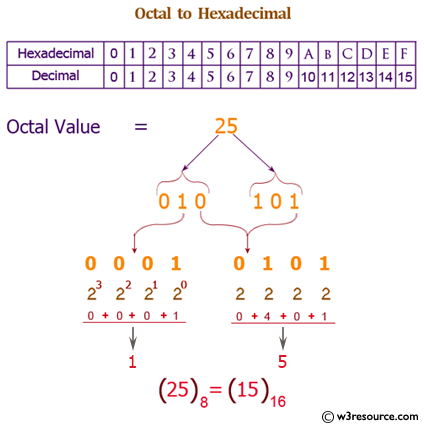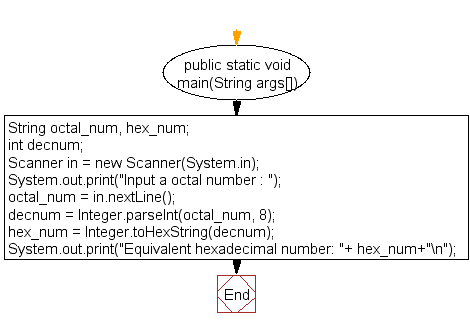﻿ Java exercises: Convert a octal number to a hexadecimal number - w3resource# Java Exercises: Convert a octal number to a hexadecimal number

## Java Basic: Exercise-27 with Solution

Write a Java program to convert a octal number to a hexadecimal number.

Octal number: The octal numeral system is the base-8 number system, and uses the digits 0 to 7.

Hexadecimal number: This is a positional numeral system with a radix, or base, of 16. Hexadecimal uses sixteen distinct symbols, most often the symbols 0-9 to represent values zero to nine, and A, B, C, D, E, F (or alternatively a, b, c, d, e, f) to represent values ten to fifteen.

Test Data:
Input any octal number: 100

Pictorial Presentation: Octal to Hexadecimal numberSample Solution:

Java Code:

``````import java.util.Scanner;

public class Exercise27 {
public static void main(String args[])
{
String octal_num, hex_num;
int decnum;
Scanner in = new Scanner(System.in);

System.out.print("Input a octal number : ");
octal_num = in.nextLine();

decnum = Integer.parseInt(octal_num, 8);
hex_num = Integer.toHexString(decnum);

}
}
```
```

Sample Output:

```Input a octal number : 100
```

Flowchart:Java Code Editor:

What is the difficulty level of this exercise?

Test your Programming skills with w3resource's quiz.

﻿

## Java: Tips of the Day

countOccurrences

Counts the occurrences of a value in an array.

Use Arrays.stream().filter().count() to count total number of values that equals the specified value.

```public static long countOccurrences(int[] numbers, int value) {
return Arrays.stream(numbers)
.filter(number -> number == value)
.count();
}
```

Ref: https://bit.ly/3kCAgLb